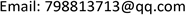MOSModeling and Simulation2324-8696Scientific Research Publishing10.12677/MOS.2021.101014MOS-40593MOS20210100000_54310203.pdf信息通讯 工程技术 不同相变材料蓄热特性及液相分数无量纲准则方程式拟合 The Heat Storage Characteristics of Different Phase Change Materials and the Liquid Phase Fraction Dimensionless Criterion Equation Fitting 宇豪1*1212上海理工大学能源与动力工程学院&amp;上海市动力工程多相流动与传热重点实验室，上海null030220211001128139© Copyright 2014 by authors and Scientific Research Publishing Inc. 2014This work is licensed under the Creative Commons Attribution International License (CC BY). http://creativecommons.org/licenses/by/4.0/The Heat Storage Characteristics of Different Phase Change Materials and the Liquid Phase Fraction Dimensionless Criterion Equation Fitting

Yuhao Zheng, Ming Zhao, Ke Wang

School of Energy and Power Engineering & Shanghai Key Laboratory of Multiphase Flow and Heat Transfer in Power Engineering, University of Shanghai for Science and Technology, ShanghaiReceived: Jan. 21st, 2021; accepted: Feb. 16th, 2021; published: Feb. 24th, 2021ABSTRACT

The main application of numerical simulation method is to study the three-tube phase change heat accumulator, which provides a reference for filling the heat accumulator with three different phase change materials (RT82, RT50, LA). Dimensionless parameters and liquid phase fitting fractions are obtained by fitting. Among them, low melting point RT50 and LA average Nusselt number have a rising stage. S t e F o R a 1 6 for different phase change materials and different heat storage methods during the melting process are obtained. Correlation function is verified by the fitting function and obtained by different Stephen number pairs, the results show that the liquid fraction function of the model has high applicability in the range of 0.0765~0.6060 of S t e ; the initial temperature below the melting point is introduced. The temperature parameter S c − 1 4 is fitted to obtain the S t e F o S c − 1 4 R a 1 6 correlation function of the liquid fraction.

Keywords:Phase Change Material, Heat Exchanger, Numerical Simulation, Liquid Fraction Fitting

Copyright © 2021 by author(s) and Hans Publishers Inc.

This work is licensed under the Creative Commons Attribution International License (CC BY 4.0).

http://creativecommons.org/licenses/by/4.0/1. 引言

2. 物理模型和数学模型2.1. 物理模型

2.2. 数学模型

G r = g β ( T w − T m ) D 3 v 2 (1)

R a = P r ⋅ G r (2)

N u ¯ = h ¯ ⋅ D k (3)

2.3. 数值计算方法和模型考核2.3.1. 数值计算方法

2.3.2. 模拟方法验证

2.3.3. 物性条件

1) 相变材料相变过程中采用层流模型，为低速非稳态不可压流动过程；

2) 相变材料和管壁材料为均质和各向同性；

3) 不考虑粘性耗散，密度采用Boussinesq假设，物性参数如表1：

Physical property parameter tabl

3. 不同相变材料的蓄热特性3.1. RT82、RT50、LA相变材料的蓄热特性

3.2. 液相对流对蓄热特性的影响

4. 液相分数的无量纲准则方程式拟合4.1. 不同相变材料液相分数拟合

S t e = C p ( T w − T m ) L (4)

Cpl为液态比热容，L为相变材料的相变潜热值，Tm为PCM的相变温度，Tw为加热表面温度。PCM液相分是函数f与无量纲参数Ste、Fo、Ra相关，其中定义变量x为 S t e F o R a 1 6 。

f = 1 − e − ( X 0.0217 ) 2 2 (5)

4.2. 不同蓄热方式液相分数拟合

1) 只有内部加热： T w = T i n = 363.15   K ， R = R m ： q = 0

2) 只有外部加热： T w = T o u t = 363.15   K ， R = R m ： q = 0

f = 1 − 1.03 e − x 0.134 (6)

f = 1 − e − ( x 0.0573 ) 2 2 (7)

4.3. 不同斯蒂芬数对拟合函数的影响

4.4. 不同初温参数对拟合函数的影响

S c = C p ( T m − T i n i t ) L (8)

Cp为比热容，L为相变材料的相变潜热值，Tm为PCM的相变温度，Tinit为初始温度。

f = 1 − e − ( x 0.04756 ) 2 2 (9)

5. 结论

1) 不同熔点的相变材料虽然自然对流强度变化存在较大差异，但存在相似的熔化阶段。

2) 对不同相变材料、内外管不同蓄热方式、不同蓄热温度拟合得到不同相变材料熔化过程的液相分数相关函数(自变量x为 S t e F o R a 1 6 )，函数准确度较好，可以预测在该模型内相变材料的液相分数变化。

3) 考虑低于熔点的初温参数对蓄热器模型的影响，通过引入初温参数 S c − 1 4 ，进过拟合得到液相分数与 S t e F o S c − 1 4 R a 1 6 相关函数，可用于预测。